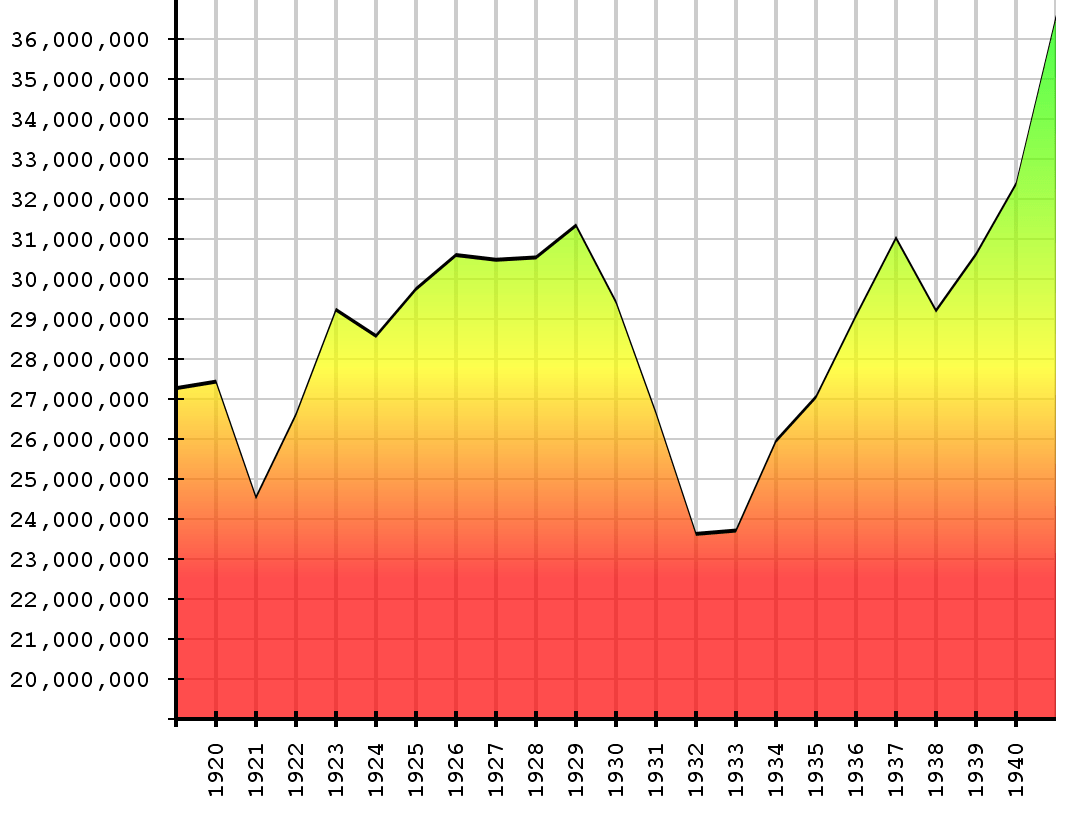## Quantity – Different Kinds of Numbers: Intervals

It is useful to look closely at this graph taken from a Wikipedia article on misleading graphs:The reason given for the graph being misleading is familiar to most of us: the labels on the vertical axis don’t start at zero, exaggerating the growth shown.   The graph, which shows employment numbers in the USA before, during, and after the Depression, makes it appear – at first glance – that the employment in 1940 is roughly three or four times what it was during the depth of the Depression in 1932-1933.   If we look at the numbers on the left, we see that employment grew from roughly 24 million to roughly 36 million, which is still very substantial, but nowhere near a tripling or quadrupling.

The reason I show you this graph is not to make any point about the vertical axis.  Instead, I want to point out that the very stricture the author of the article about misleading graphs wants us to get – that axes should start at zero – very obviously does not apply to the horizontal axis.  Nobody has ever seriously suggested that graphs should start with year 0 A.D.

The kind of number we use for measuring time is a different kind of number than the one we use for measuring employment.  It is not simply that the units are different: people (or millions of people) as the unit for employment, and years as the unit for time.   There is something else going on when we talk about 1940 as the value for the time.  It isn’t sufficient to say that 1940 is the number of years, to be precise we would have to say 1940 years since a particular reference moment.  In our calendar, the reference moment is the year of the birth of Jesus of Nazareth, as we have reconstructed it to the best of our ability.  Wikipedia mentions that the A.D. scale wasn’t used until the year 525.

For the name for the kind of number, like time, where the precise location of zero is essentially arbitrary, we can use interval.   Though the number 2000 is twice the number 1000, there is little sense in claiming that 2000 A.D. is twice 1000 A.D.  Those two moments in time (that is, June 4, 2000 and June 4, 1000) have different numbers associated with them in the Jewish calendar (5760, 4760).  In each of those calendars, the difference between the two numbers will be 1000.  The ratios between the numbers will be different.  2000/1000 = 2; 5760/4760 = 1.21.  It is sometimes said that ratios are meaningless for interval numbers, but that is overstating it: ratios are perfectly meaningful for differences.  From 1940 to 1980 is twice the stretch of years (40) as 2000 to 2020; this observation would hold true if you converted it to Jewish calendar years as well.  Despite different dates for New Year’s day, and different rules for leap years etc., both calendars have years that average about 365¼ days.

We usually think of 1980, 1940 and their difference, 40, as all being expressed in the same units: years.  I’m suggesting that this is somewhat misleading, as it obscures the difference in the kind of number each represents.  The 40 years difference between 1980 and 1940 is not an interval kind of number.  1980 years since the beginning of the Common Era – 1940 years since the beginning of the Common Era = 40 years.

Temperature scales, whether Fahrenheit or Celsius (centigrade) are also interval numbers.  The zero point on each scale is different, and both are somewhat arbitrary.  As it turns out, temperature can in fact be measured on a scale that is not an interval scale.  It is called absolute temperature, and it is based on an absolute zero: the temperature at which all motion ceases.  The Kelvin temperature scale uses this absolute zero.  Differences between temperatures on the Kelvin scale are the same as differences between temperatures on the Celsius scale.  Kelvin temperatures have values that are 273 higher than the corresponding temperatures on the Celsius scale.  “Absolute zero” temperature is measured as -273 degrees on the Celsius scale.

Distances are measured in miles or meters or other units; but miles are also often used as interval numbers.   An Interstate near where I live has its exits marked in miles.  The mile numbers correspond to the distance of the exit from the beginning of the Interstate.  Coordinate systems also mark points with values measured in distance units; these are almost always interval kinds of numbers since the origin (the point labeled zero) of the coordinate system is almost always arbitrary.   Labeling a point as “1 mile east and 2 miles north” works fine as long as we agree on the starting point, the reference point.

The differences between years and “years-since”, or miles and “miles-east-of”, are usually ignored or glossed over in school settings.  I think that – mostly – this is completely defensible.  It becomes an issue for most students only when they start to deal with a subject called “transformation of coordinate systems” somewhere in ninth grade or so.  Yet, even then, neither text books nor teachers tend to clearly distinguish between interval kinds of numbers and multiplicative unit kinds of numbers.

This entry was posted in Uncategorized and tagged , , , , . Bookmark the permalink.